Want to share your content on R-bloggers? click here if you have a blog, or here if you don't.

In my last post, I analysed how the level of education is affected by region, sex and year. In this post, I will continue with the same dataset but this time I will include age in the analysis. Please send suggestions for improvement of the analysis to .

First, define libraries and functions.

``library (tidyverse)``
``## -- Attaching packages -------------------------------------------------- tidyverse 1.3.0 --``
``````## v ggplot2 3.2.1     v purrr   0.3.3
## v tibble  2.1.3     v dplyr   0.8.3
## v tidyr   1.0.2     v stringr 1.4.0
## v readr   1.3.1     v forcats 0.4.0``````
``````## -- Conflicts ----------------------------------------------------- tidyverse_conflicts() --
``````library (broom)
library (car)``````
``## Loading required package: carData``
``````##
## Attaching package: 'car'``````
``````## The following object is masked from 'package:dplyr':
##
##     recode``````
``````## The following object is masked from 'package:purrr':
##
##     some``````
``library (sjPlot)``
``````## Registered S3 methods overwritten by 'lme4':
##   method                          from
##   cooks.distance.influence.merMod car
##   influence.merMod                car
##   dfbeta.influence.merMod         car
##   dfbetas.influence.merMod        car``````
``````library (leaps)
library (MASS)``````
``````##
## Attaching package: 'MASS'``````
``````## The following object is masked from 'package:dplyr':
##
##     select``````
``library (earth)``
``## Warning: package 'earth' was built under R version 3.6.3``
``## Loading required package: Formula``
``## Loading required package: plotmo``
``## Warning: package 'plotmo' was built under R version 3.6.3``
``## Loading required package: plotrix``
``## Loading required package: TeachingDemos``
``## Warning: package 'TeachingDemos' was built under R version 3.6.3``
``library (acepack)``
``## Warning: package 'acepack' was built under R version 3.6.3``
``library (lspline)``
``## Warning: package 'lspline' was built under R version 3.6.3``
``library (lme4)``
``## Loading required package: Matrix``
``````##
## Attaching package: 'Matrix'``````
``````## The following objects are masked from 'package:tidyr':
##
##     expand, pack, unpack``````
``library (pROC)``
``## Warning: package 'pROC' was built under R version 3.6.3``
``## Type 'citation("pROC")' for a citation.``
``````##
## Attaching package: 'pROC'``````
``````## The following objects are masked from 'package:stats':
##
##     cov, smooth, var``````
``library (boot)``
``````##
## Attaching package: 'boot'``````
``````## The following object is masked from 'package:car':
##
##     logit``````
``library (faraway)``
``````##
## Attaching package: 'faraway'``````
``````## The following objects are masked from 'package:boot':
##
##     logit, melanoma``````
``````## The following objects are masked from 'package:car':
##
##     logit, vif``````
``library (arm)``
``## Warning: package 'arm' was built under R version 3.6.3``
``````##
## arm (Version 1.10-1, built: 2018-4-12)``````
``## Working directory is C:/R/rblog/content/post``
``````##
## Attaching package: 'arm'``````
``````## The following objects are masked from 'package:faraway':
##
##     fround, logit, pfround``````
``````## The following object is masked from 'package:boot':
##
##     logit``````
``````## The following object is masked from 'package:plotrix':
##
##     rescale``````
``````## The following object is masked from 'package:car':
##
##     logit``````
``````readfile <- function (file1){read_csv (file1, col_types = cols(), locale = readr::locale (encoding = "latin1"), na = c("..", "NA")) %>%
gather (starts_with("19"), starts_with("20"), key = "year", value = groupsize) %>%
drop_na() %>%
mutate (year_n = parse_number (year))
}

perc_women <- function(x){
ifelse (length(x) == 2, x / (x + x), NA)
}

mutate(NUTS2_sh = substr(NUTS2, 3, 4))``````

The data table is downloaded from Statistics Sweden. It is saved as a comma-delimited file without heading, UF0506A1.csv, http://www.statistikdatabasen.scb.se/pxweb/en/ssd/.

I will calculate the percentage of women in for the different education levels in the different regions for each year. In my later analysis, I will use the number of people in each education level, region, year and age.

The table: Population 16-74 years of age by region, highest level of education, age and sex. Year 1985 – 2018 NUTS 2 level 2008- 10 year intervals (16-74)

The data is aggregated, the size of each group is in the column groupsize.

``````tb <- readfile("UF0506A1.csv") %>%
mutate(edulevel = `level of education`) %>%
group_by(edulevel, region, year, sex, age) %>%
mutate(groupsize_age = sum(groupsize)) %>%
group_by(edulevel, region, year, age) %>%
mutate (sum_edu_region_year = sum(groupsize)) %>%
mutate (perc_women = perc_women (groupsize_age)) %>%
group_by(region, year) %>%
mutate (sum_pop = sum(groupsize)) %>% rowwise() %>%
mutate(age_l = unlist(lapply(strsplit(substr(age, 1, 5), "-"), strtoi))) %>%
rowwise() %>%
mutate(age_h = unlist(lapply(strsplit(substr(age, 1, 5), "-"), strtoi))) %>%
mutate(age_n = (age_l + age_h) / 2) %>%
left_join(nuts %>% distinct (NUTS2_en, NUTS2_sh), by = c("region" = "NUTS2_en"))``````
``````## Warning: Column `region`/`NUTS2_en` joining character vector and factor,
## coercing into character vector``````
``````numedulevel <- read.csv("edulevel_1.csv")

numedulevel[, 2] <- data.frame(c(8, 9, 10, 12, 13, 15, 22, NA))

numedulevel %>%
knitr::kable(
booktabs = TRUE,
caption = 'Calculated in previous post, length of education') ``````
Table 1: Calculated in previous post, length of education
level.of.educationeduyears
primary and secondary education less than 9 years (ISCED97 1)8
primary and secondary education 9-10 years (ISCED97 2)9
upper secondary education, 2 years or less (ISCED97 3C)10
upper secondary education 3 years (ISCED97 3A)12
post-secondary education, less than 3 years (ISCED97 4+5B)13
post-secondary education 3 years or more (ISCED97 5A)15
no information about level of educational attainmentNA
``````tbnum <- tb %>%
right_join(numedulevel, by = c("level of education" = "level.of.education")) %>%
filter(!is.na(eduyears)) %>%
drop_na()``````
``````## Warning: Column `level of education`/`level.of.education` joining character
## vector and factor, coercing into character vector``````
``````tbnum %>%
filter (year_n == 2018) %>%
ggplot () +
geom_point (mapping = aes(x = NUTS2_sh,y = perc_women, colour = age_n)) +
facet_grid(. ~ eduyears)``````Figure 1: Population by region, level of education, percent women and year, Year 1985 – 2018

``````tbnum %>%
filter (year_n == 2008) %>%
ggplot () +
geom_point (mapping = aes(x = NUTS2_sh,y = perc_women, colour = age_n)) +
facet_grid(. ~ eduyears)``````Figure 2: Population by region, level of education, percent women and year, Year 1985 – 2018

``````tbnum %>%
filter (year_n == 1998) %>%
ggplot () +
geom_point (mapping = aes(x = NUTS2_sh,y = perc_women, colour = age_n)) +
facet_grid(. ~ eduyears)``````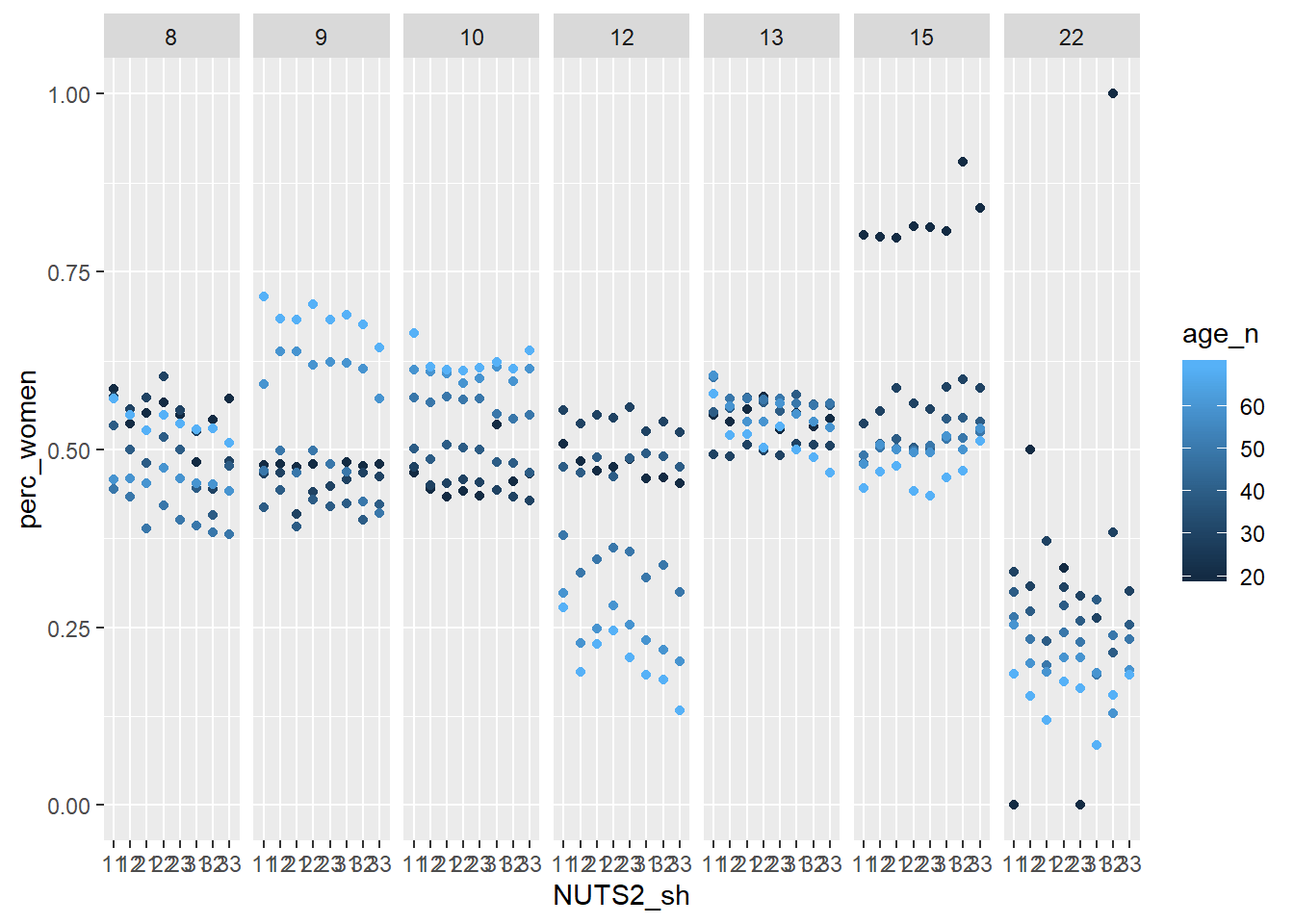Figure 3: Population by region, level of education, percent women and year, Year 1985 – 2018

``````tbnum %>%
filter (year_n == 1988) %>%
ggplot () +
geom_point (mapping = aes(x = NUTS2_sh,y = perc_women, colour = age_n)) +
facet_grid(. ~ eduyears)``````Figure 4: Population by region, level of education, percent women and year, Year 1985 – 2018

``summary(tbnum)``
``````##     region              age            level of education     sex
##  Length:22660       Length:22660       Length:22660       Length:22660
##  Class :character   Class :character   Class :character   Class :character
##  Mode  :character   Mode  :character   Mode  :character   Mode  :character
##
##
##
##      year             groupsize         year_n       edulevel
##  Length:22660       Min.   :    0   Min.   :1985   Length:22660
##  Class :character   1st Qu.: 1713   1st Qu.:1993   Class :character
##  Mode  :character   Median : 5737   Median :2001   Mode  :character
##                     Mean   : 9638   Mean   :2001
##                     3rd Qu.:14135   3rd Qu.:2010
##                     Max.   :77163   Max.   :2018
##  groupsize_age   sum_edu_region_year   perc_women        sum_pop
##  Min.   :    0   Min.   :     1      Min.   :0.0000   Min.   : 266057
##  1st Qu.: 1713   1st Qu.:  3566      1st Qu.:0.4090   1st Qu.: 560119
##  Median : 5737   Median : 11566      Median :0.4875   Median : 859794
##  Mean   : 9638   Mean   : 19276      Mean   :0.4707   Mean   : 824996
##  3rd Qu.:14135   3rd Qu.: 28287      3rd Qu.:0.5542   3rd Qu.:1200038
##  Max.   :77163   Max.   :134645      Max.   :1.0000   Max.   :1716160
##      age_l           age_h           age_n         NUTS2_sh
##  Min.   :16.00   Min.   :24.00   Min.   :20.00   Length:22660
##  1st Qu.:25.00   1st Qu.:34.00   1st Qu.:29.50   Class :character
##  Median :45.00   Median :54.00   Median :49.50   Mode  :character
##  Mean   :40.37   Mean   :49.21   Mean   :44.79
##  3rd Qu.:55.00   3rd Qu.:64.00   3rd Qu.:59.50
##  Max.   :65.00   Max.   :74.00   Max.   :69.50
##     eduyears
##  Min.   : 8.00
##  1st Qu.: 9.00
##  Median :12.00
##  Mean   :12.64
##  3rd Qu.:15.00
##  Max.   :22.00``````

Let’s investigate the shape of the function for the response and predictors. The shape of the predictors has a great impact on the rest of the analysis. I use acepack to fit a model and plot both the response and the predictors.

``````tbtest <- tbnum %>% dplyr::select(sum_pop, sum_edu_region_year, year_n, perc_women, age_n)

tbtest <- data.frame(tbtest)

acefit <- ace(tbtest, tbnum\$eduyears, wt=tbtest\$sum_edu_region_year)

plot(tbnum\$eduyears, acefit\$ty, xlab = "Years of education", ylab = "transformed years of education")``````Figure 5: Plots of the response and predictors using acepack

``plot(tbtest[,1], acefit\$tx[,1], xlab = "Population in region", ylab = "transformed population in region")``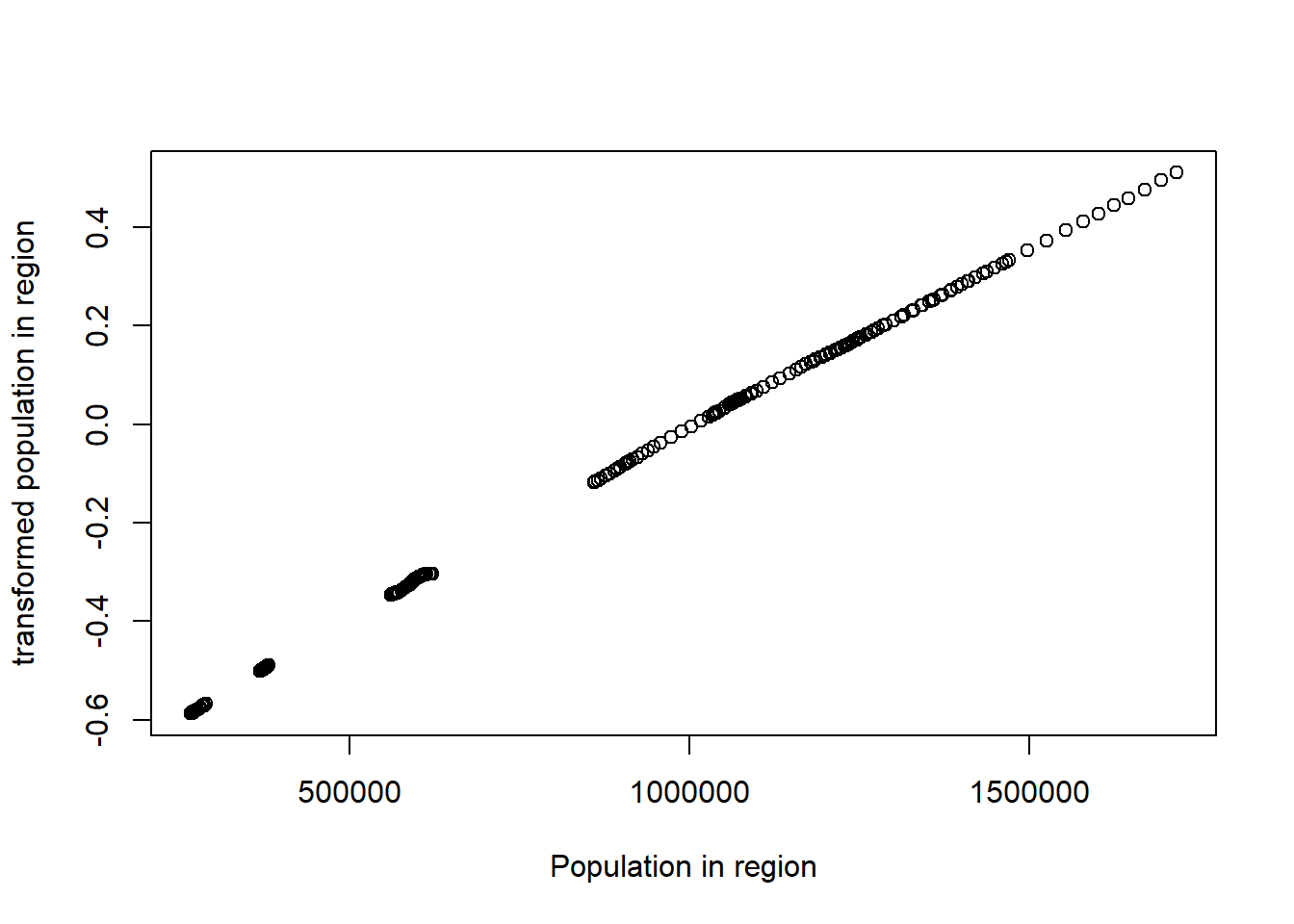Figure 6: Plots of the response and predictors using acepack

``plot(tbtest[,2], acefit\$tx[,2], xlab = "# persons with same edulevel, region, year", ylab = "transformed # persons with same edulevel, region, year")``Figure 7: Plots of the response and predictors using acepack

``plot(tbtest[,3], acefit\$tx[,3], xlab = "Year", ylab = "transformed year")``Figure 8: Plots of the response and predictors using acepack

``plot(tbtest[,4], acefit\$tx[,4], xlab = "Percent women", ylab = "transformed percent women")``Figure 9: Plots of the response and predictors using acepack

``plot(tbtest[,5], acefit\$tx[,5], xlab = "Age", ylab = "transformed age")``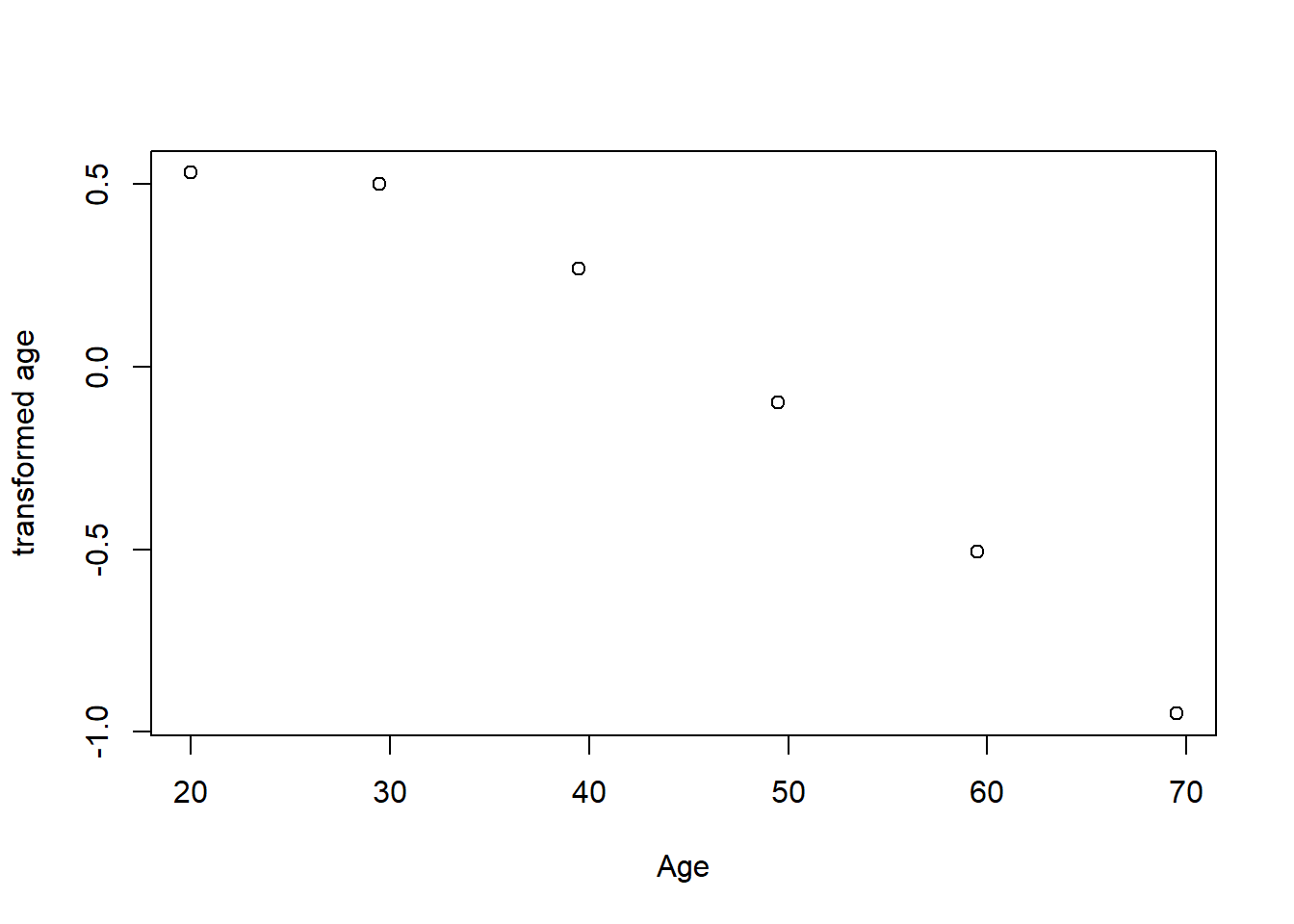Figure 10: Plots of the response and predictors using acepack

I use MARS to fit hockey-stick functions for the predictors. I do not wish to overfit by using a better approximation at this point. I will include interactions of degree two. I will put more emphasis on groups with larger size by using the number of persons with same edulevel, region, year, age as weights in the regression. From the analysis with acepack, I will approximate the shape of the response with X^-1.

``````tbtemp <- tbnum %>% dplyr::select(eduyears, sum_pop, sum_edu_region_year, year_n, perc_women, age_n)

mmod <- earth(eduyears^-1 ~ ., weights = tbtest\$sum_edu_region_year, data = tbtemp, nk = 9, degree = 2)

summary (mmod)``````
``````## Call: earth(formula=eduyears^-1~., data=tbtemp,
##             weights=tbtest\$sum_edu_region_year, degree=2, nk=9)
##
##                                         coefficients
## (Intercept)                              0.109974110
## h(68923-sum_edu_region_year)            -0.000000172
## h(sum_edu_region_year-68923)            -0.000000071
## h(0.402156-perc_women)                  -0.142039538
## h(perc_women-0.402156)                  -0.216582584
## h(2004-year_n) * h(perc_women-0.402156)  0.006151369
## h(year_n-2004) * h(perc_women-0.402156) -0.003086559
## h(perc_women-0.402156) * h(age_n-29.5)   0.004789032
## h(perc_women-0.402156) * h(29.5-age_n)   0.007923485
##
## Selected 9 of 9 terms, and 4 of 5 predictors
## Termination condition: Reached nk 9
## Importance: perc_women, age_n, year_n, sum_edu_region_year, sum_pop-unused
## Weights: 160, 160, 2503, 2503, 27984, 27984, 41596, 41596, 57813, 57813,...
## Number of terms at each degree of interaction: 1 4 4
## GCV 4.263981    RSS 96442.81    GRSq 0.3866042    RSq 0.3876866``````
``plot (mmod)``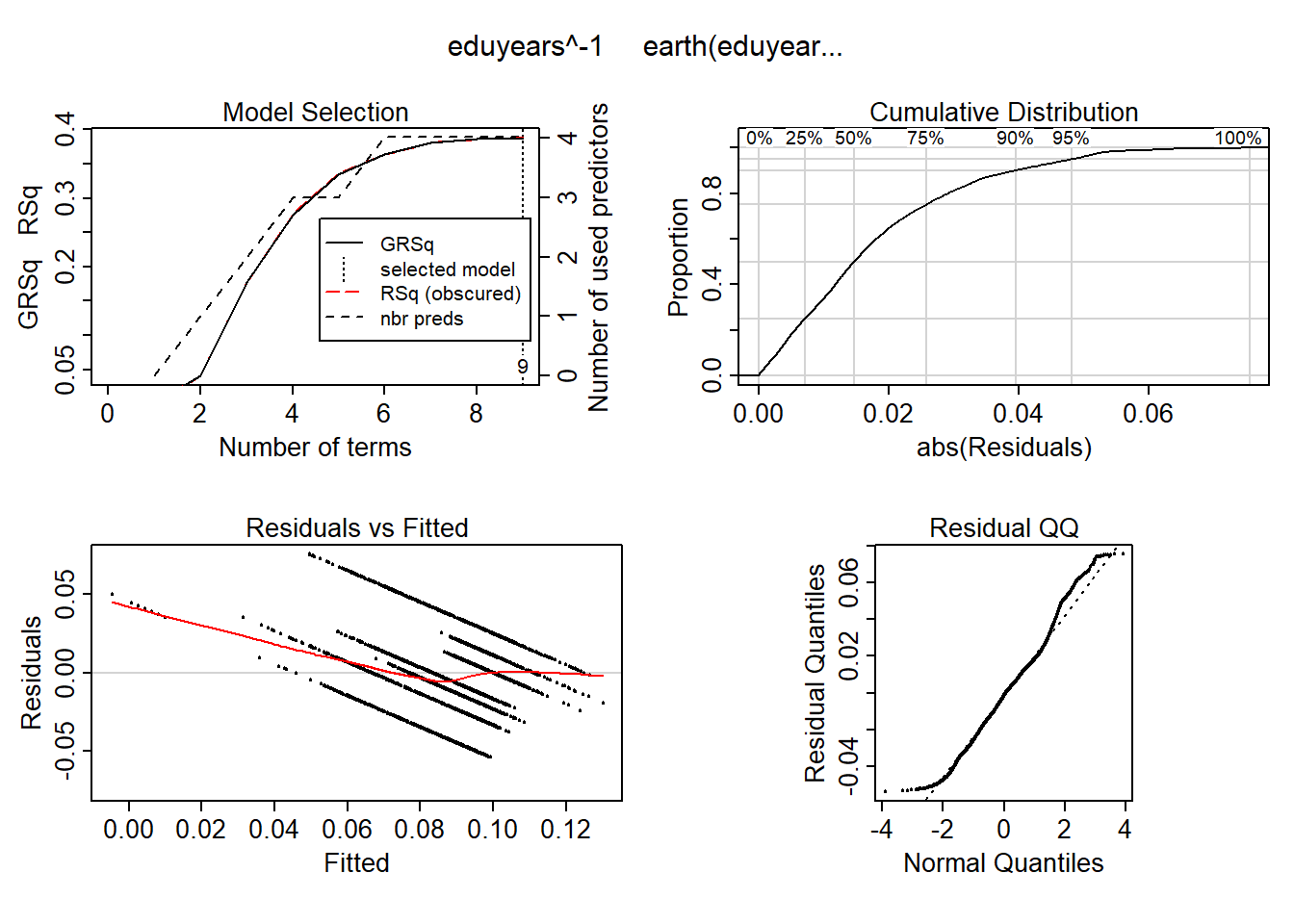Figure 11: Hockey-stick functions fit with MARS for the predictors, Year 1985 – 2018

``plotmo (mmod)``
``````##  plotmo grid:    sum_pop sum_edu_region_year year_n perc_women age_n
##                   859794             11566.5   2001  0.4875054  49.5``````Figure 12: Hockey-stick functions fit with MARS for the predictors, Year 1985 – 2018

``````model_mmod <- lm (eduyears^-1 ~
lspline(sum_edu_region_year, c(68923)) +
lspline(perc_women, c(0.402156)) +
lspline(year_n, c(2004)):lspline(perc_women, c(0.402156)) +
lspline(perc_women, c(0.402156)):lspline(age_n, c(29.5)),
weights = tbtest\$sum_edu_region_year,
data = tbnum)

summary (model_mmod)\$r.squared``````
``##  0.4067181``
``anova (model_mmod)``
``````## Analysis of Variance Table
##
## Response: eduyears^-1
##                                                              Df Sum Sq Mean Sq
## lspline(sum_edu_region_year, c(68923))                        2   6429  3214.4
## lspline(perc_women, c(0.402156))                              2  11182  5590.8
## lspline(perc_women, c(0.402156)):lspline(year_n, c(2004))     4  18631  4657.7
## lspline(perc_women, c(0.402156)):lspline(age_n, c(29.5))      4  27819  6954.8
## Residuals                                                 22647  93445     4.1
##                                                           F value    Pr(>F)
## lspline(sum_edu_region_year, c(68923))                     779.03 < 2.2e-16 ***
## lspline(perc_women, c(0.402156))                          1354.96 < 2.2e-16 ***
## lspline(perc_women, c(0.402156)):lspline(year_n, c(2004)) 1128.81 < 2.2e-16 ***
## lspline(perc_women, c(0.402156)):lspline(age_n, c(29.5))  1685.54 < 2.2e-16 ***
## Residuals
## ---
## Signif. codes:  0 '***' 0.001 '**' 0.01 '*' 0.05 '.' 0.1 ' ' 1``````

Since the predictors are on different scales I will also use MARS to fit hockey-stick functions to the standardised data. Weights can not be negative.

``````tbtemp <- tbnum %>% dplyr::select(eduyears, sum_pop, sum_edu_region_year, year_n, perc_women, age_n)

tbtemp_scale <- data.frame(tbtemp %>% scale())

mmod_scale <- earth(eduyears^-1 ~ ., weights = tbnum\$sum_edu_region_year, data = tbtemp_scale, nk = 9, degree = 2)

summary (mmod_scale)``````
``````## Call: earth(formula=eduyears^-1~., data=tbtemp_scale,
##             weights=tbnum\$sum_edu_region_year, degree=2, nk=9)
##
##                                coefficients
## (Intercept)                       1.4518768
## h(1.54673-sum_pop)               -1.3123162
## h(2.48479-sum_edu_region_year)    2.2482786
## h(sum_edu_region_year-2.48479)    0.4668398
## h(0.589918-perc_women)           -5.1021696
## h(perc_women-0.589918)           -5.8909808
## h(-0.905736-age_n)               -3.0330857
## h(age_n- -0.905736)              -0.5385299
##
## Selected 8 of 9 terms, and 4 of 5 predictors
## Termination condition: Reached nk 9
## Importance: perc_women, sum_edu_region_year, sum_pop, age_n, year_n-unused
## Weights: 160, 160, 2503, 2503, 27984, 27984, 41596, 41596, 57813, 57813,...
## Number of terms at each degree of interaction: 1 7 (additive model)
## GCV 388811.6    RSS 8796089602    GRSq 0.2645814    RSq 0.2657169``````
``plot (mmod_scale)``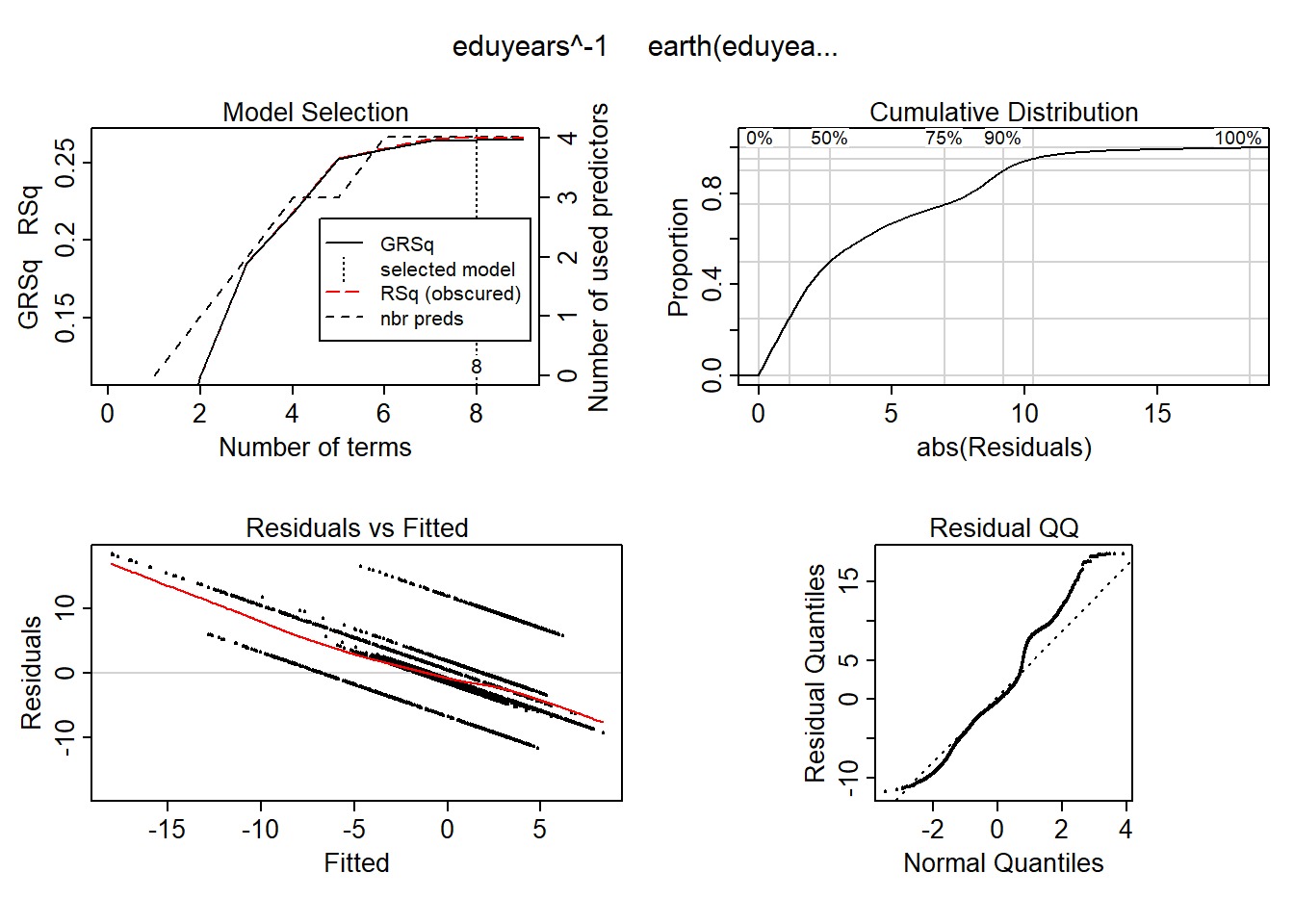Figure 13: Hockey-stick functions fit with MARS for the predictors, Year 1985 – 2018

``plotmo (mmod_scale)``
``````##  plotmo grid:    sum_pop sum_edu_region_year      year_n perc_women     age_n
##               0.08635757          -0.3683495 -0.04979415  0.1299828 0.2792169``````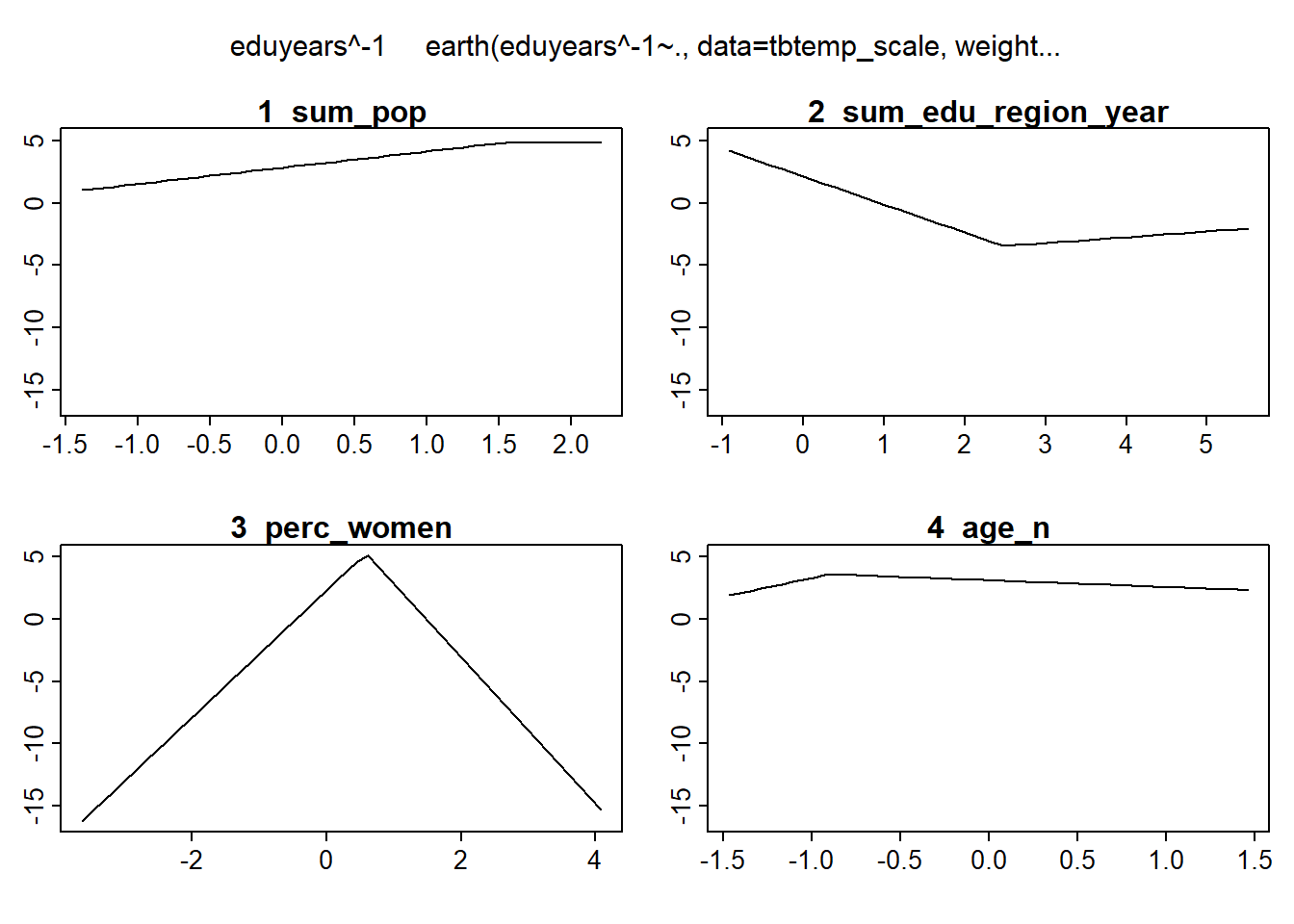Figure 14: Hockey-stick functions fit with MARS for the predictors, Year 1985 – 2018

``````model_mmod_scale <- lm (eduyears^-1 ~
lspline(sum_pop, c(1.54673)) +
lspline(sum_edu_region_year, c(2.48479)) +
lspline(perc_women, c(0.589918)) +
lspline(age_n, c(-0.905736)),
weights = tbtest\$sum_edu_region_year,
data = tbtemp_scale)

summary (model_mmod_scale)\$r.squared``````
``##  0.2657429``
``anova (model_mmod_scale)``
``````## Analysis of Variance Table
##
## Response: eduyears^-1
##                                             Df     Sum Sq    Mean Sq  F value
## lspline(sum_pop, c(1.54673))                 2   22976857   11488429   29.585
## lspline(sum_edu_region_year, c(2.48479))     2  824771527  412385764 1061.981
## lspline(perc_women, c(0.589918))             2 2195580032 1097790016 2827.043
## lspline(age_n, c(-0.905736))                 2  140046090   70023045  180.324
## Residuals                                22651 8795778088     388317
##                                             Pr(>F)
## lspline(sum_pop, c(1.54673))             1.473e-13 ***
## lspline(sum_edu_region_year, c(2.48479)) < 2.2e-16 ***
## lspline(perc_women, c(0.589918))         < 2.2e-16 ***
## lspline(age_n, c(-0.905736))             < 2.2e-16 ***
## Residuals
## ---
## Signif. codes:  0 '***' 0.001 '**' 0.01 '*' 0.05 '.' 0.1 ' ' 1``````

I will use regsubsets to find the model which minimises the AIC using the standardised data. I will include some plots for diagnostic purposes. I have also included a bootstrap test since we can’t count on the errors to be normally distributed.

``````b <- regsubsets (eduyears^-1 ~ (lspline(sum_edu_region_year, c(2.48479)) + lspline(perc_women, c(0.589918)) + lspline(age_n, c(-0.905736)) + year_n + lspline(sum_pop, c(1.54673))) * (lspline(sum_edu_region_year, c(2.48479)) + lspline(perc_women, c(0.589918)) + lspline(age_n, c(-0.905736)) + year_n + lspline(sum_pop, c(1.54673))), weights = tbnum\$sum_edu_region_year, data = tbtemp_scale, nvmax = 12)

rs <- summary(b)
AIC <- 50 * log (rs\$rss / 50) + (2:13) * 2
which.min (AIC)``````
``##  4``
``names (rs\$which[4,])[rs\$which[4,]]``
``````##  "(Intercept)"
##  "lspline(perc_women, c(0.589918))1"
##  "lspline(sum_edu_region_year, c(2.48479))1:lspline(age_n, c(-0.905736))1"
##  "lspline(perc_women, c(0.589918))2:lspline(age_n, c(-0.905736))2"
##  "lspline(age_n, c(-0.905736))1:lspline(sum_pop, c(1.54673))1"``````
``````model <- lm (eduyears^-1 ~
lspline(perc_women, c(0.402156)) +
lspline(sum_edu_region_year, c(68923)):lspline(age_n, c(29.5)) +
lspline(perc_women, c(0.402156)):lspline(age_n, c(29.5)) +
lspline(age_n, c(29.5)):sum_pop,
weights = tbnum\$sum_edu_region_year,
data = tbnum)

boxcox(model)``````Figure 15: Find the model that minimises the AIC, Year 1985 – 2018

``summary (model)``
``````##
## Call:
## lm(formula = eduyears^-1 ~ lspline(perc_women, c(0.402156)) +
##     lspline(sum_edu_region_year, c(68923)):lspline(age_n, c(29.5)) +
##     lspline(perc_women, c(0.402156)):lspline(age_n, c(29.5)) +
##     lspline(age_n, c(29.5)):sum_pop, data = tbnum, weights = tbnum\$sum_edu_region_year)
##
## Weighted Residuals:
##     Min      1Q  Median      3Q     Max
## -7.7236 -1.3350 -0.2622  1.2344  6.2808
##
## Coefficients:
##                                                                    Estimate
## (Intercept)                                                       6.003e-02
## lspline(perc_women, c(0.402156))1                                 1.614e-01
## lspline(perc_women, c(0.402156))2                                -9.712e-02
## lspline(sum_edu_region_year, c(68923))1:lspline(age_n, c(29.5))1  1.680e-09
## lspline(sum_edu_region_year, c(68923))2:lspline(age_n, c(29.5))1 -9.445e-10
## lspline(sum_edu_region_year, c(68923))1:lspline(age_n, c(29.5))2  1.449e-08
## lspline(sum_edu_region_year, c(68923))2:lspline(age_n, c(29.5))2 -1.359e-10
## lspline(perc_women, c(0.402156))1:lspline(age_n, c(29.5))1       -1.611e-03
## lspline(perc_women, c(0.402156))2:lspline(age_n, c(29.5))1       -2.570e-03
## lspline(perc_women, c(0.402156))1:lspline(age_n, c(29.5))2        1.802e-04
## lspline(perc_women, c(0.402156))2:lspline(age_n, c(29.5))2        3.535e-03
## lspline(age_n, c(29.5))1:sum_pop                                 -2.162e-10
## lspline(age_n, c(29.5))2:sum_pop                                 -3.873e-10
##                                                                  Std. Error
## (Intercept)                                                       1.629e-03
## lspline(perc_women, c(0.402156))1                                 6.578e-03
## lspline(perc_women, c(0.402156))2                                 2.087e-02
## lspline(sum_edu_region_year, c(68923))1:lspline(age_n, c(29.5))1  3.435e-10
## lspline(sum_edu_region_year, c(68923))2:lspline(age_n, c(29.5))1  6.753e-10
## lspline(sum_edu_region_year, c(68923))1:lspline(age_n, c(29.5))2  4.415e-10
## lspline(sum_edu_region_year, c(68923))2:lspline(age_n, c(29.5))2  1.118e-09
## lspline(perc_women, c(0.402156))1:lspline(age_n, c(29.5))1        1.893e-04
## lspline(perc_women, c(0.402156))2:lspline(age_n, c(29.5))1        7.417e-04
## lspline(perc_women, c(0.402156))1:lspline(age_n, c(29.5))2        6.717e-05
## lspline(perc_women, c(0.402156))2:lspline(age_n, c(29.5))2        1.212e-04
## lspline(age_n, c(29.5))1:sum_pop                                  1.854e-11
## lspline(age_n, c(29.5))2:sum_pop                                  2.233e-11
##                                                                  t value
## (Intercept)                                                       36.838
## lspline(perc_women, c(0.402156))1                                 24.541
## lspline(perc_women, c(0.402156))2                                 -4.653
## lspline(sum_edu_region_year, c(68923))1:lspline(age_n, c(29.5))1   4.890
## lspline(sum_edu_region_year, c(68923))2:lspline(age_n, c(29.5))1  -1.399
## lspline(sum_edu_region_year, c(68923))1:lspline(age_n, c(29.5))2  32.817
## lspline(sum_edu_region_year, c(68923))2:lspline(age_n, c(29.5))2  -0.122
## lspline(perc_women, c(0.402156))1:lspline(age_n, c(29.5))1        -8.510
## lspline(perc_women, c(0.402156))2:lspline(age_n, c(29.5))1        -3.465
## lspline(perc_women, c(0.402156))1:lspline(age_n, c(29.5))2         2.683
## lspline(perc_women, c(0.402156))2:lspline(age_n, c(29.5))2        29.180
## lspline(age_n, c(29.5))1:sum_pop                                 -11.660
## lspline(age_n, c(29.5))2:sum_pop                                 -17.345
##                                                                  Pr(>|t|)
## (Intercept)                                                       < 2e-16 ***
## lspline(perc_women, c(0.402156))1                                 < 2e-16 ***
## lspline(perc_women, c(0.402156))2                                3.30e-06 ***
## lspline(sum_edu_region_year, c(68923))1:lspline(age_n, c(29.5))1 1.01e-06 ***
## lspline(sum_edu_region_year, c(68923))2:lspline(age_n, c(29.5))1 0.161945
## lspline(sum_edu_region_year, c(68923))1:lspline(age_n, c(29.5))2  < 2e-16 ***
## lspline(sum_edu_region_year, c(68923))2:lspline(age_n, c(29.5))2 0.903193
## lspline(perc_women, c(0.402156))1:lspline(age_n, c(29.5))1        < 2e-16 ***
## lspline(perc_women, c(0.402156))2:lspline(age_n, c(29.5))1       0.000531 ***
## lspline(perc_women, c(0.402156))1:lspline(age_n, c(29.5))2       0.007309 **
## lspline(perc_women, c(0.402156))2:lspline(age_n, c(29.5))2        < 2e-16 ***
## lspline(age_n, c(29.5))1:sum_pop                                  < 2e-16 ***
## lspline(age_n, c(29.5))2:sum_pop                                  < 2e-16 ***
## ---
## Signif. codes:  0 '***' 0.001 '**' 0.01 '*' 0.05 '.' 0.1 ' ' 1
##
## Residual standard error: 2.069 on 22647 degrees of freedom
## Multiple R-squared:  0.3847, Adjusted R-squared:  0.3844
## F-statistic:  1180 on 12 and 22647 DF,  p-value: < 2.2e-16``````
``anova (model)``
``````## Analysis of Variance Table
##
## Response: eduyears^-1
##                                                                   Df Sum Sq
## lspline(perc_women, c(0.402156))                                   2  13795
## lspline(sum_edu_region_year, c(68923)):lspline(age_n, c(29.5))     4  29033
## lspline(perc_women, c(0.402156)):lspline(age_n, c(29.5))           4   8736
## lspline(age_n, c(29.5)):sum_pop                                    2   9027
## Residuals                                                      22647  96916
##                                                                Mean Sq F value
## lspline(perc_women, c(0.402156))                                6897.3 1611.75
## lspline(sum_edu_region_year, c(68923)):lspline(age_n, c(29.5))  7258.3 1696.10
## lspline(perc_women, c(0.402156)):lspline(age_n, c(29.5))        2183.9  510.33
## lspline(age_n, c(29.5)):sum_pop                                 4513.4 1054.67
## Residuals                                                          4.3
##                                                                   Pr(>F)
## lspline(perc_women, c(0.402156))                               < 2.2e-16 ***
## lspline(sum_edu_region_year, c(68923)):lspline(age_n, c(29.5)) < 2.2e-16 ***
## lspline(perc_women, c(0.402156)):lspline(age_n, c(29.5))       < 2.2e-16 ***
## lspline(age_n, c(29.5)):sum_pop                                < 2.2e-16 ***
## Residuals
## ---
## Signif. codes:  0 '***' 0.001 '**' 0.01 '*' 0.05 '.' 0.1 ' ' 1``````
``plot (model)``Figure 16: Find the model that minimises the AIC, Year 1985 – 2018Figure 17: Find the model that minimises the AIC, Year 1985 – 2018Figure 18: Find the model that minimises the AIC, Year 1985 – 2018Figure 19: Find the model that minimises the AIC, Year 1985 – 2018

``binnedplot(predict(model), resid(model))``Figure 20: Find the model that minimises the AIC, Year 1985 – 2018

``halfnorm(rstudent(model))``Figure 21: Find the model that minimises the AIC, Year 1985 – 2018

``````tbnum %>% mutate(residuals = residuals(model)) %>%
group_by(eduyears, region, year_n, age_n) %>%
summarise(residuals = mean(residuals), count = sum(groupsize)) %>%
ggplot (aes(x = eduyears, y = residuals, size = sqrt(count), colour = year_n)) +
geom_point() + facet_grid(. ~ age_n)``````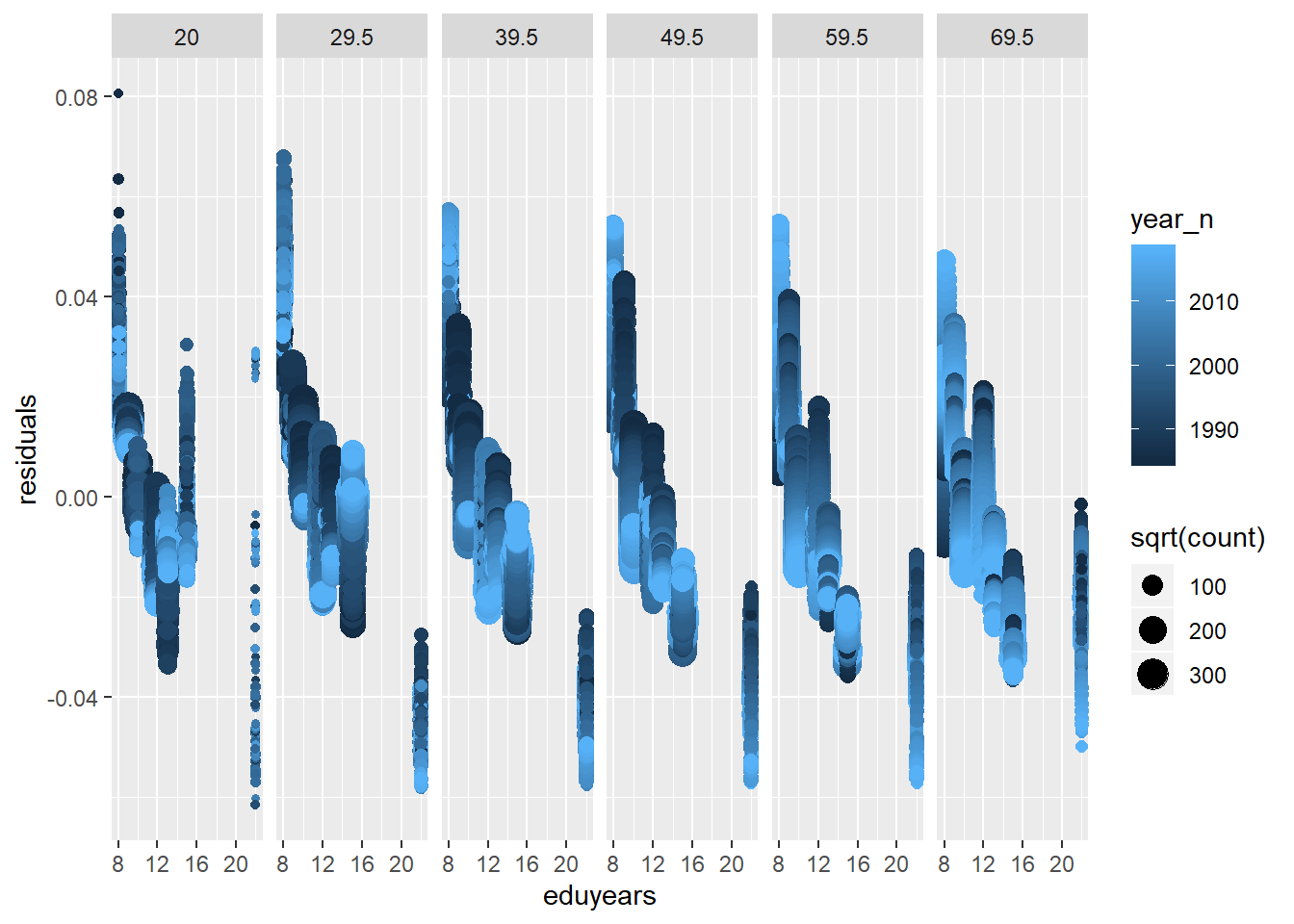Figure 22: Find the model that minimises the AIC, Year 1985 – 2018

``````tbnumpred <- bind_cols(tbnum, as_tibble(predict(model, tbnum, interval = "confidence")))

suppressWarnings(multiclass.roc(tbnumpred\$eduyears, tbnumpred\$fit))``````
``## Setting direction: controls > cases``
``## Setting direction: controls < cases``
``````## Setting direction: controls > cases
## Setting direction: controls > cases
## Setting direction: controls > cases
## Setting direction: controls > cases``````
``## Setting direction: controls < cases``
``````## Setting direction: controls > cases
## Setting direction: controls > cases
## Setting direction: controls > cases
## Setting direction: controls > cases
## Setting direction: controls > cases
## Setting direction: controls > cases
## Setting direction: controls > cases
## Setting direction: controls > cases
## Setting direction: controls > cases
## Setting direction: controls > cases
## Setting direction: controls > cases
## Setting direction: controls > cases
## Setting direction: controls > cases
## Setting direction: controls > cases``````
``````##
## Call:
## multiclass.roc.default(response = tbnumpred\$eduyears, predictor = tbnumpred\$fit)
##
## Data: tbnumpred\$fit with 7 levels of tbnumpred\$eduyears: 8, 9, 10, 12, 13, 15, 22.
## Multi-class area under the curve: 0.6936``````
``````bs <- function(formula, data, indices) {
d <- data[indices,] # allows boot to select sample
fit <- lm(formula, weights = sum_edu_region_year, data=d)
return(coef(fit))
}

results <- boot(data = tbnum, statistic = bs,
R = 1000, formula = eduyears^-1 ~
lspline(perc_women, c(0.402156)) +
lspline(sum_edu_region_year, c(68923)):lspline(age_n, c(29.5)) +
lspline(perc_women, c(0.402156)):lspline(age_n, c(29.5)) +
lspline(age_n, c(29.5)):sum_pop,
weights = tbnum\$sum_edu_region_year)

results``````
``````##
## WEIGHTED BOOTSTRAP
##
##
## Call:
## boot(data = tbnum, statistic = bs, R = 1000, weights = tbnum\$sum_edu_region_year,
##     formula = eduyears^-1 ~ lspline(perc_women, c(0.402156)) +
##         lspline(sum_edu_region_year, c(68923)):lspline(age_n,
##             c(29.5)) + lspline(perc_women, c(0.402156)):lspline(age_n,
##         c(29.5)) + lspline(age_n, c(29.5)):sum_pop)
##
##
## Bootstrap Statistics :
##           original        bias     std. error      mean(t*)
## t1*   6.002560e-02  6.282966e-03 1.487621e-03  6.630857e-02
## t2*   1.614448e-01 -1.489491e-02 6.393635e-03  1.465499e-01
## t3*  -9.712093e-02  7.221993e-02 1.927104e-02 -2.490101e-02
## t4*   1.679729e-09  4.440291e-09 3.270648e-10  6.120020e-09
## t5*  -9.444671e-10  1.440855e-10 3.744453e-10 -8.003816e-10
## t6*   1.448852e-08 -3.952505e-09 4.193252e-10  1.053602e-08
## t7*  -1.359400e-10  1.468111e-09 5.529310e-10  1.332171e-09
## t8*  -1.610831e-03  6.882804e-05 1.801159e-04 -1.542003e-03
## t9*  -2.570250e-03 -2.067477e-03 6.732789e-04 -4.637727e-03
## t10*  1.801919e-04  7.229534e-04 6.282813e-05  9.031453e-04
## t11*  3.535199e-03 -1.161393e-03 1.113341e-04  2.373805e-03
## t12* -2.161738e-10 -2.930531e-10 1.634625e-11 -5.092269e-10
## t13* -3.872669e-10  6.108726e-11 2.103217e-11 -3.261797e-10``````
``plot(results, index=1) # intercept ``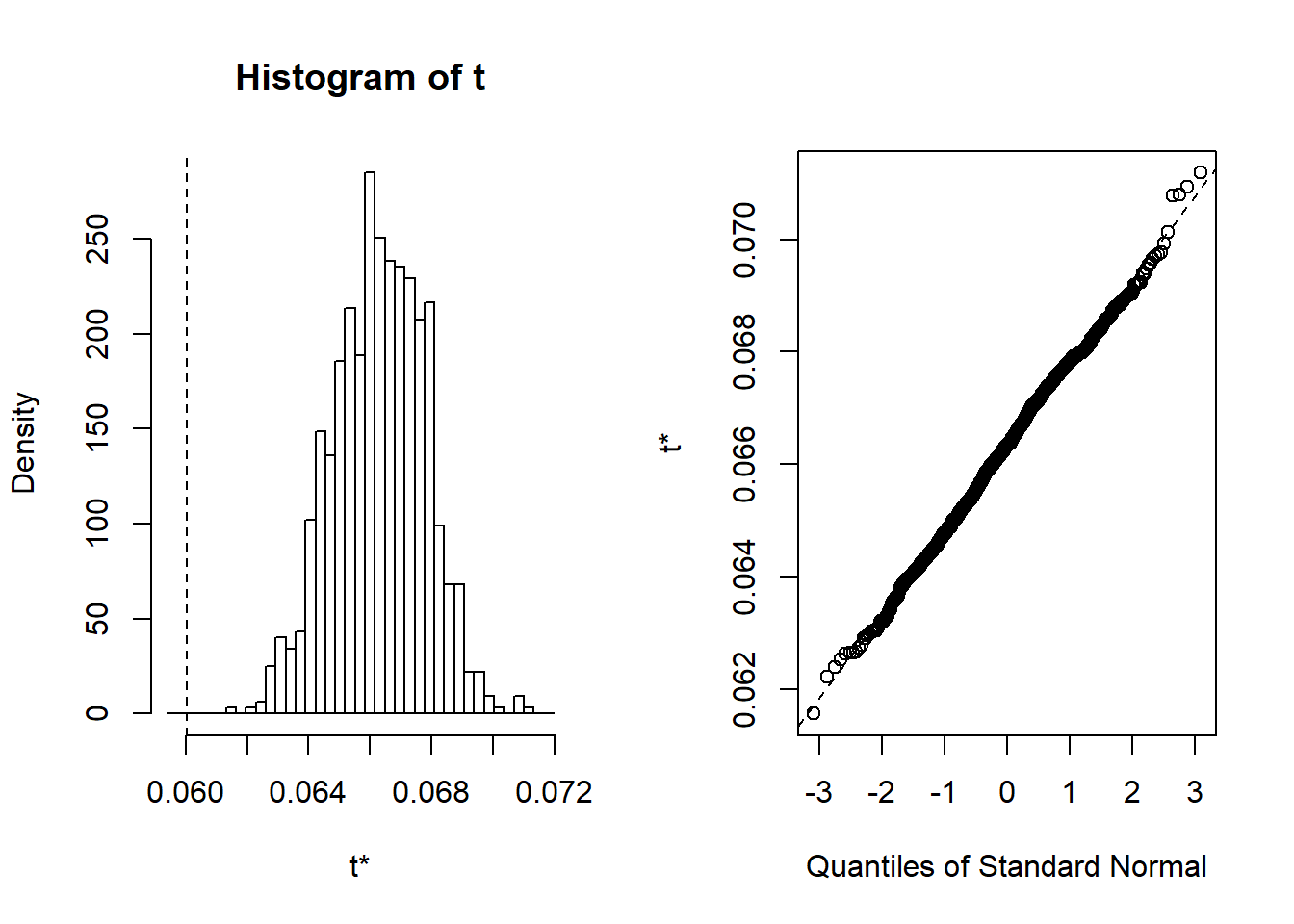Figure 23: Find the model that minimises the AIC, Year 1985 – 2018

There are a few things I would like to investigate to improve the credibility of the analysis. I will assume that the data can be grouped by year of birth and region and investigate how this will affect the model. I have scaled the weights not to affect the residual standard deviation. I will include some plots for diagnostic purposes. I will assume that each year of birth and each region is a group and set them as random effects and the rest of the predictors as fixed effects. I use the mean age in each age group as the year of birth.

``````temp <- tbnum %>% mutate(yob = year_n - age_n) %>% mutate(region = tbnum\$region)

temp <- data.frame(temp)

weights_scaled <- tbtest\$sum_edu_region_year / max(tbtest\$sum_edu_region_year)

mmodel <- lmer (eduyears^-1 ~
lspline(perc_women, c(0.402156)) +
lspline(sum_edu_region_year, c(68923)):lspline(age_n, c(29.5)) +
lspline(perc_women, c(0.402156)):lspline(age_n, c(29.5)) +
lspline(age_n, c(29.5)):sum_pop +
(1|yob) +
(1|region),
weights = weights_scaled,
data = temp) ``````
``````## Warning: Some predictor variables are on very different scales: consider
## rescaling``````
``plot(mmodel)``Figure 24: A diagnostic plot of the model with random effects components

``qqnorm (residuals(mmodel), main="")``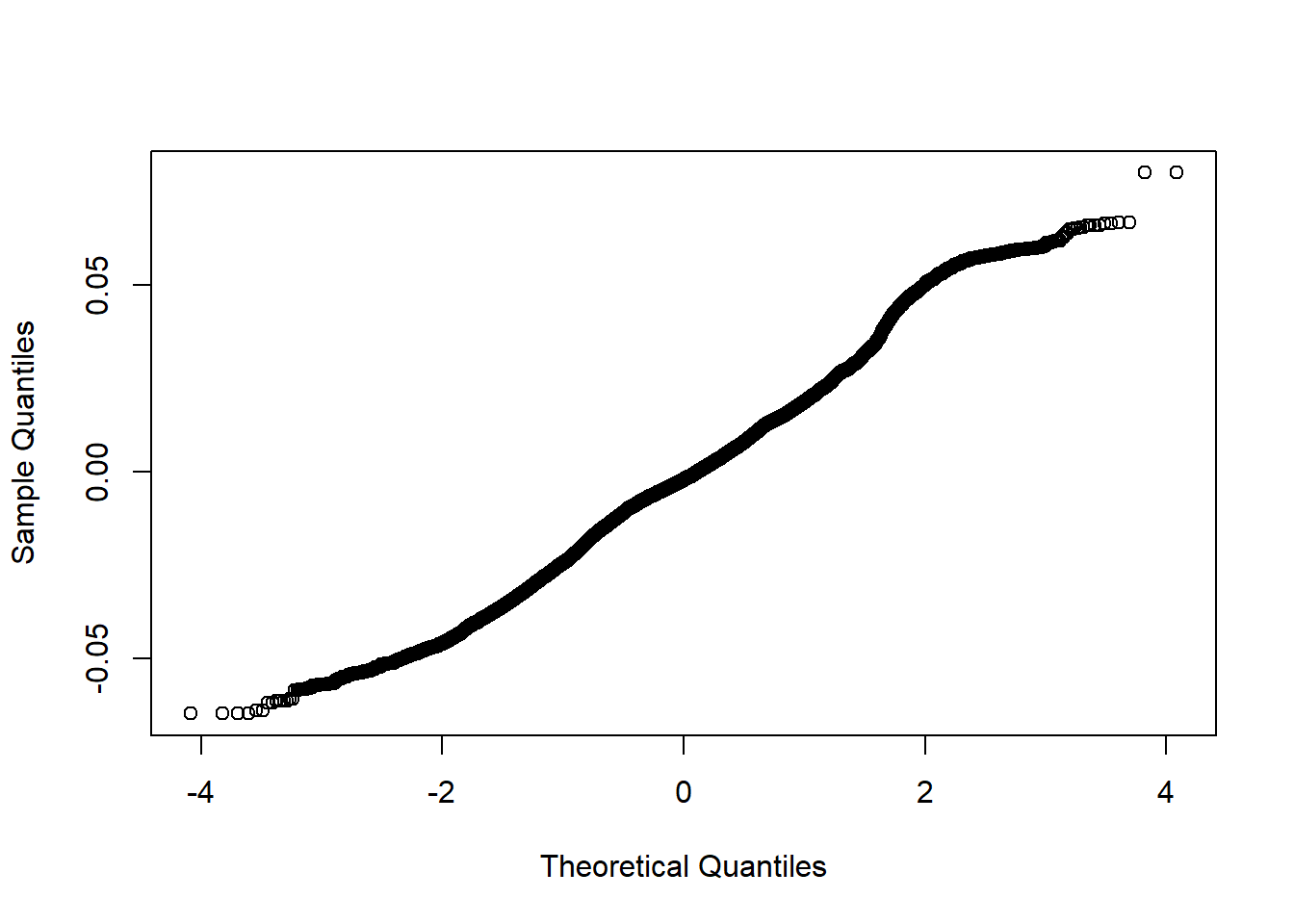Figure 25: A diagnostic plot of the model with random effects components

``summary (mmodel)``
``````## Linear mixed model fit by REML ['lmerMod']
## Formula:
## eduyears^-1 ~ lspline(perc_women, c(0.402156)) + lspline(sum_edu_region_year,
##     c(68923)):lspline(age_n, c(29.5)) + lspline(perc_women, c(0.402156)):lspline(age_n,
##     c(29.5)) + lspline(age_n, c(29.5)):sum_pop + (1 | yob) +      (1 | region)
##    Data: temp
## Weights: weights_scaled
##
## REML criterion at convergence: -107912.7
##
## Scaled residuals:
##     Min      1Q  Median      3Q     Max
## -4.1769 -0.6147 -0.1003  0.6108  3.2800
##
## Random effects:
##  Groups   Name        Variance  Std.Dev.
##  yob      (Intercept) 1.053e-04 0.0102603
##  region   (Intercept) 8.113e-07 0.0009007
##  Residual             2.753e-05 0.0052472
## Number of obs: 22660, groups:  yob, 108; region, 8
##
## Fixed effects:
##                                                                    Estimate
## (Intercept)                                                       3.382e-02
## lspline(perc_women, c(0.402156))1                                 1.806e-01
## lspline(perc_women, c(0.402156))2                                -1.222e-01
## lspline(sum_edu_region_year, c(68923))1:lspline(age_n, c(29.5))1  3.692e-10
## lspline(sum_edu_region_year, c(68923))2:lspline(age_n, c(29.5))1  1.706e-09
## lspline(sum_edu_region_year, c(68923))1:lspline(age_n, c(29.5))2  1.440e-08
## lspline(sum_edu_region_year, c(68923))2:lspline(age_n, c(29.5))2 -5.056e-09
## lspline(perc_women, c(0.402156))1:lspline(age_n, c(29.5))1        8.518e-04
## lspline(perc_women, c(0.402156))2:lspline(age_n, c(29.5))1       -1.818e-03
## lspline(perc_women, c(0.402156))1:lspline(age_n, c(29.5))2       -1.046e-03
## lspline(perc_women, c(0.402156))2:lspline(age_n, c(29.5))2        2.786e-03
## lspline(age_n, c(29.5))1:sum_pop                                 -1.412e-10
## lspline(age_n, c(29.5))2:sum_pop                                 -3.426e-10
##                                                                  Std. Error
## (Intercept)                                                       2.092e-03
## lspline(perc_women, c(0.402156))1                                 1.757e-02
## lspline(perc_women, c(0.402156))2                                 1.953e-02
## lspline(sum_edu_region_year, c(68923))1:lspline(age_n, c(29.5))1  3.244e-10
## lspline(sum_edu_region_year, c(68923))2:lspline(age_n, c(29.5))1  6.564e-10
## lspline(sum_edu_region_year, c(68923))1:lspline(age_n, c(29.5))2  4.148e-10
## lspline(sum_edu_region_year, c(68923))2:lspline(age_n, c(29.5))2  1.112e-09
## lspline(perc_women, c(0.402156))1:lspline(age_n, c(29.5))1        5.885e-04
## lspline(perc_women, c(0.402156))2:lspline(age_n, c(29.5))1        6.941e-04
## lspline(perc_women, c(0.402156))1:lspline(age_n, c(29.5))2        6.737e-05
## lspline(perc_women, c(0.402156))2:lspline(age_n, c(29.5))2        1.167e-04
## lspline(age_n, c(29.5))1:sum_pop                                  3.051e-11
## lspline(age_n, c(29.5))2:sum_pop                                  2.143e-11
##                                                                  t value
## (Intercept)                                                       16.167
## lspline(perc_women, c(0.402156))1                                 10.280
## lspline(perc_women, c(0.402156))2                                 -6.258
## lspline(sum_edu_region_year, c(68923))1:lspline(age_n, c(29.5))1   1.138
## lspline(sum_edu_region_year, c(68923))2:lspline(age_n, c(29.5))1   2.599
## lspline(sum_edu_region_year, c(68923))1:lspline(age_n, c(29.5))2  34.716
## lspline(sum_edu_region_year, c(68923))2:lspline(age_n, c(29.5))2  -4.549
## lspline(perc_women, c(0.402156))1:lspline(age_n, c(29.5))1         1.448
## lspline(perc_women, c(0.402156))2:lspline(age_n, c(29.5))1        -2.619
## lspline(perc_women, c(0.402156))1:lspline(age_n, c(29.5))2       -15.525
## lspline(perc_women, c(0.402156))2:lspline(age_n, c(29.5))2        23.876
## lspline(age_n, c(29.5))1:sum_pop                                  -4.627
## lspline(age_n, c(29.5))2:sum_pop                                 -15.987``````
``````##
## Correlation matrix not shown by default, as p = 13 > 12.
## Use print(x, correlation=TRUE)  or
##     vcov(x)        if you need it``````
``````## fit warnings:
## Some predictor variables are on very different scales: consider rescaling``````
``anova (mmodel)``
``````## Analysis of Variance Table
##                                                                Df   Sum Sq
## lspline(perc_women, c(0.402156))                                2 0.243294
## lspline(sum_edu_region_year, c(68923)):lspline(age_n, c(29.5))  4 0.060730
## lspline(perc_women, c(0.402156)):lspline(age_n, c(29.5))        4 0.030402
## lspline(age_n, c(29.5)):sum_pop                                 2 0.014060
##                                                                 Mean Sq F value
## lspline(perc_women, c(0.402156))                               0.121647 4418.15
## lspline(sum_edu_region_year, c(68923)):lspline(age_n, c(29.5)) 0.015182  551.42
## lspline(perc_women, c(0.402156)):lspline(age_n, c(29.5))       0.007600  276.04
## lspline(age_n, c(29.5)):sum_pop                                0.007030  255.32``````
``binnedplot(predict(mmodel), resid(mmodel))``Figure 26: A diagnostic plot of the model with random effects components

``halfnorm(rstudent(mmodel))``Figure 27: A diagnostic plot of the model with random effects components

``````tbnum %>% mutate(residuals = residuals(mmodel)) %>%
group_by(eduyears, region, year_n, age_n) %>%
summarise(residuals = mean(residuals), count = sum(groupsize)) %>%
ggplot (aes(x = eduyears, y = residuals, size = sqrt(count), colour = year_n)) +
geom_point() + facet_grid(. ~ age_n)``````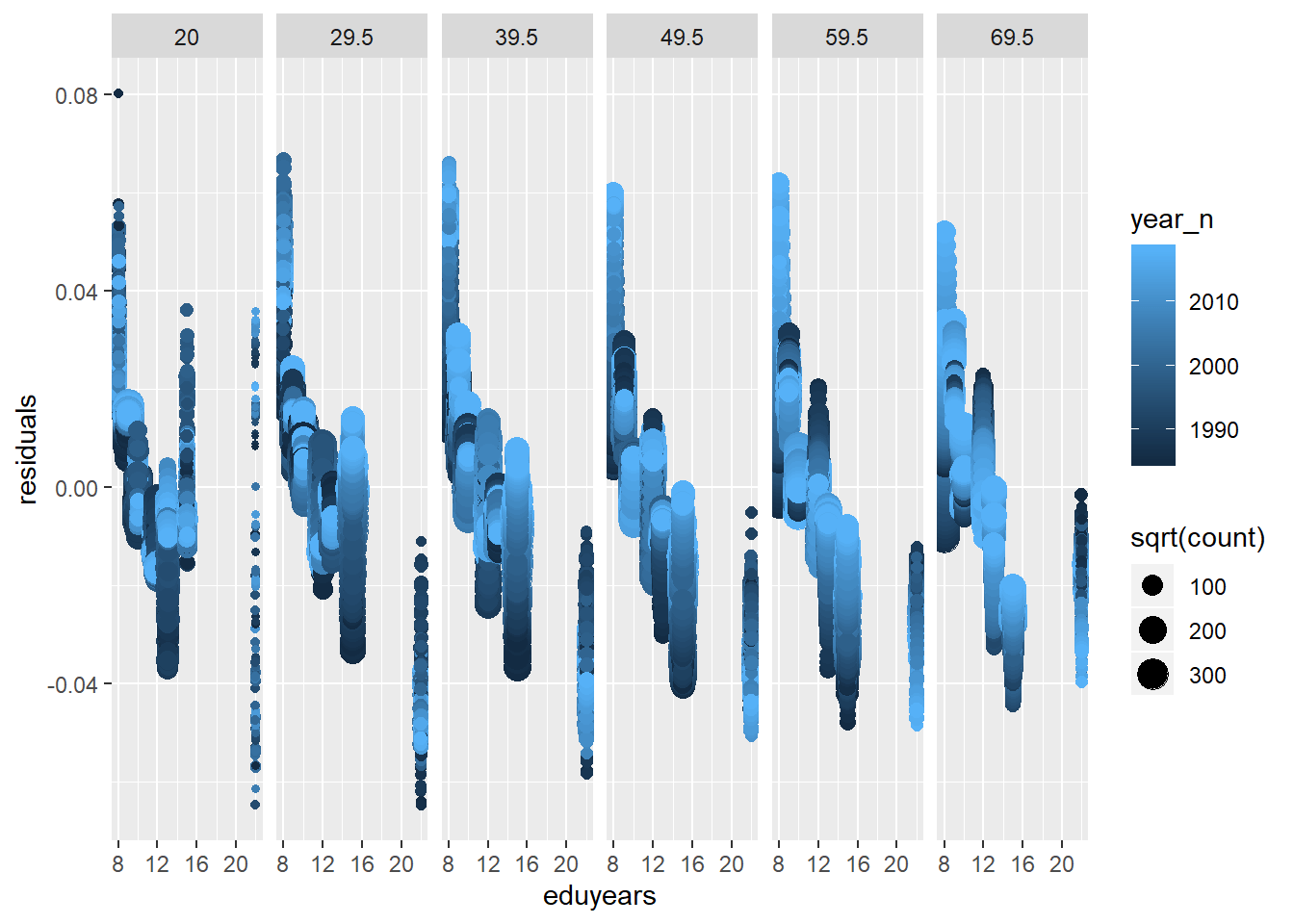Figure 28: A diagnostic plot of the model with random effects components

``tbnumpred <- bind_cols(temp, as_tibble(predict(mmodel, temp, interval = "confidence")))``
``````## Warning in predict.merMod(mmodel, temp, interval = "confidence"): unused
## arguments ignored``````
``````## Warning: Calling `as_tibble()` on a vector is discouraged, because the behavior is likely to change in the future. Use `tibble::enframe(name = NULL)` instead.
## This warning is displayed once per session.``````
``suppressWarnings (multiclass.roc (tbnumpred\$eduyears, tbnumpred\$value))``
``## Setting direction: controls > cases``
``## Setting direction: controls < cases``
``````## Setting direction: controls > cases
## Setting direction: controls > cases
## Setting direction: controls > cases
## Setting direction: controls > cases``````
``## Setting direction: controls < cases``
``````## Setting direction: controls > cases
## Setting direction: controls > cases
## Setting direction: controls > cases
## Setting direction: controls > cases
## Setting direction: controls > cases
## Setting direction: controls > cases
## Setting direction: controls > cases
## Setting direction: controls > cases
## Setting direction: controls > cases
## Setting direction: controls > cases
## Setting direction: controls > cases``````
``## Setting direction: controls < cases``
``````## Setting direction: controls > cases
## Setting direction: controls > cases``````
``````##
## Call:
## multiclass.roc.default(response = tbnumpred\$eduyears, predictor = tbnumpred\$value)
##
## Data: tbnumpred\$value with 7 levels of tbnumpred\$eduyears: 8, 9, 10, 12, 13, 15, 22.
## Multi-class area under the curve: 0.6939``````
``````mySumm <- function(.) { s <- sigma(.)
c(beta =getME(., "beta"), sigma = s, sig01 = unname(s * getME(., "theta"))) }

results <- bootMer(mmodel, mySumm, nsim = 1000)

results``````
``````##
## PARAMETRIC BOOTSTRAP
##
##
## Call:
## bootMer(x = mmodel, FUN = mySumm, nsim = 1000)
##
##
## Bootstrap Statistics :
##           original        bias     std. error
## t1*   3.382171e-02  3.204383e-05 1.370011e-03
## t2*   1.806110e-01  5.656583e-04 1.586369e-02
## t3*  -1.222301e-01 -1.200144e-04 7.545495e-03
## t4*   3.692235e-10  1.574681e-11 1.758515e-10
## t5*   1.705945e-09 -8.046354e-12 5.355611e-10
## t6*   1.440064e-08 -1.696450e-11 2.268515e-10
## t7*  -5.055769e-09  1.234462e-11 8.717959e-10
## t8*   8.518246e-04 -2.117494e-05 5.396222e-04
## t9*  -1.817991e-03  5.758882e-06 2.800208e-04
## t10* -1.045953e-03  1.165115e-06 3.192411e-05
## t11*  2.786456e-03 -8.257204e-08 6.151912e-05
## t12* -1.411911e-10 -7.934184e-13 2.229494e-11
## t13* -3.426241e-10  1.956803e-13 9.642223e-12
## t14*  5.247231e-03 -3.268970e-03 1.377226e-05
## t15*  1.026026e-02 -6.487712e-06 6.957391e-04
## t16*  9.007187e-04 -1.190123e-05 2.607631e-04``````
``````##
## 118 warning(s): Model failed to converge with max|grad| = 0.00200096 (tol = 0.002, component 1) (and others)``````
``plot(results)``Figure 29: A diagnostic plot of the model with random effects components

Now let’s see what we have found. I will plot all the models for comparison. I could not get the back transformation in sjPlot to work for the response variable so you will have to endure that the response is the inverse of years of education.

``````transformeddata <- tbnum %>%  mutate(eduyears = eduyears ^ -1)

model <- lm (eduyears ~
lspline(perc_women, c(0.402156)) +
lspline(sum_edu_region_year, c(68923)):lspline(age_n, c(29.5)) +
lspline(perc_women, c(0.402156)):lspline(age_n, c(29.5)) +
lspline(age_n, c(29.5)):sum_pop,
weights = tbnum\$sum_edu_region_year,
data = transformeddata)

temp <- transformeddata %>% mutate(yob = year_n - age_n) %>% mutate(region = tbnum\$region)

temp <- data.frame(temp)

mmodel <- lmer (eduyears ~
lspline(perc_women, c(0.402156)) +
lspline(sum_edu_region_year, c(68923)):lspline(age_n, c(29.5)) +
lspline(perc_women, c(0.402156)):lspline(age_n, c(29.5)) +
lspline(age_n, c(29.5)):sum_pop +
(1|yob) +
(1|region),
weights = weights_scaled,
data = temp) ``````
``````## Warning: Some predictor variables are on very different scales: consider
## rescaling``````
``plot_model (model, type = "pred", terms = c("perc_women"))``Figure 30: The significance of the per cent women on the level of education, Year 1985 – 2018

``plot_model (mmodel, type = "pred", terms = c("perc_women"))``Figure 31: The significance of the per cent women on the level of education, Year 1985 – 2018

``````tbnum %>%
ggplot () +
geom_jitter (mapping = aes(x = perc_women, y = eduyears )) +
labs(
x = "Per cent women",
y = "Years of education"
)``````Figure 32: The significance of the per cent women on the level of education, Year 1985 – 2018

``plot_model (model, type = "pred", terms = c("age_n", "sum_edu_region_year"))``Figure 33: The significance of the interaction between the number of persons with the same level of education, region and year, age and age on the level of education, Year 1985 – 2018

``plot_model (mmodel, type = "pred", terms = c("age_n", "sum_edu_region_year"))``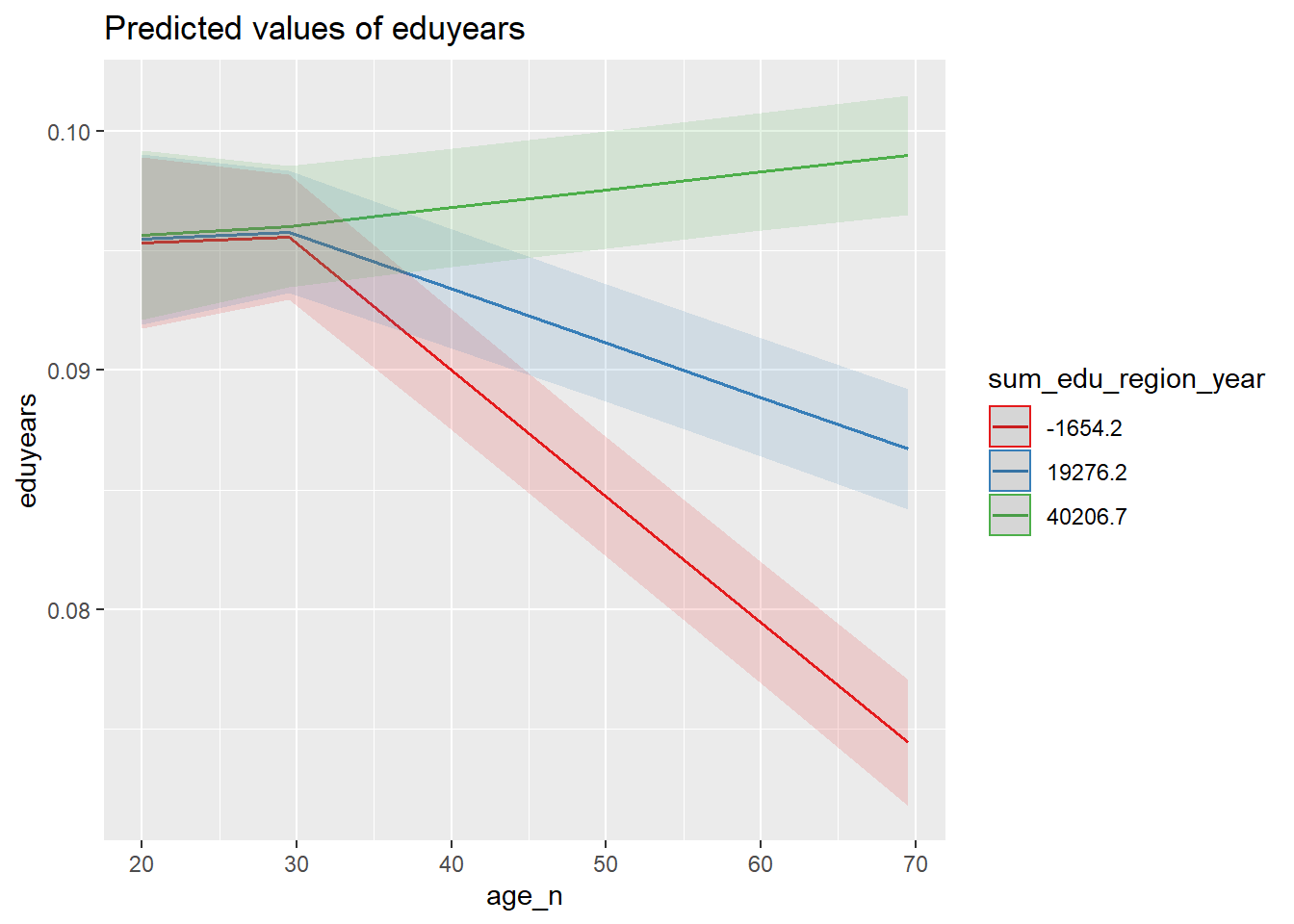Figure 34: The significance of the interaction between the number of persons with the same level of education, region and year, age and age on the level of education, Year 1985 – 2018

``````tbnum %>%
ggplot () +
geom_jitter (mapping = aes(x = sum_edu_region_year, y = eduyears, colour = age_n)) +
labs(
x = "# persons with same edulevel, region, year, age",
y = "Years of education"
)``````Figure 35: The significance of the interaction between the number of persons with the same level of education, region and year, age and age on the level of education, Year 1985 – 2018

``plot_model (model, type = "pred", terms = c("age_n", "perc_women"))``Figure 36: The significance of the interaction between per cent women and age on the level of education, Year 1985 – 2018

``plot_model (mmodel, type = "pred", terms = c("age_n", "perc_women"))``Figure 37: The significance of the interaction between per cent women and age on the level of education, Year 1985 – 2018

``````tbnum %>%
ggplot () +
geom_jitter (mapping = aes(x = perc_women, y = eduyears, colour = age_n)) +
labs(
x = "Age",
y = "Years of education"
)``````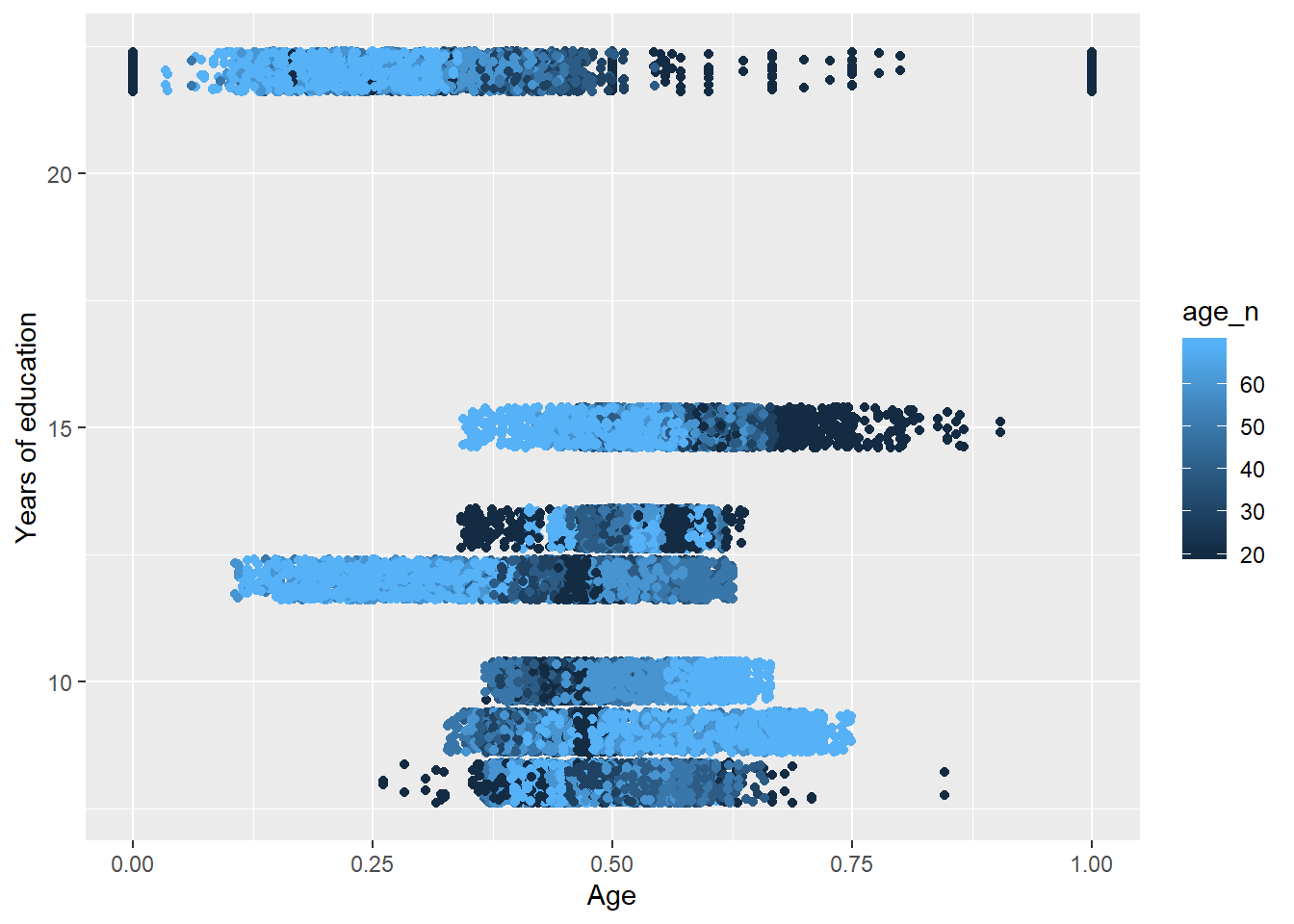Figure 38: The significance of the interaction between per cent women and age on the level of education, Year 1985 – 2018

``plot_model (model, type = "pred", terms = c("age_n", "sum_pop"))``Figure 39: The significance of the interaction between the age and population in the region on the level of education, Year 1985 – 2018

``plot_model (mmodel, type = "pred", terms = c("age_n", "sum_pop"))``Figure 40: The significance of the interaction between the age and population in the region on the level of education, Year 1985 – 2018

``````tbnum %>%
ggplot () +
geom_jitter (mapping = aes(x = age_n, y = eduyears, colour = sum_pop)) +
labs(
x = "Age",
y = "Years of education"
)``````Figure 41: The significance of the interaction between the age and population in the region on the level of education, Year 1985 – 2018